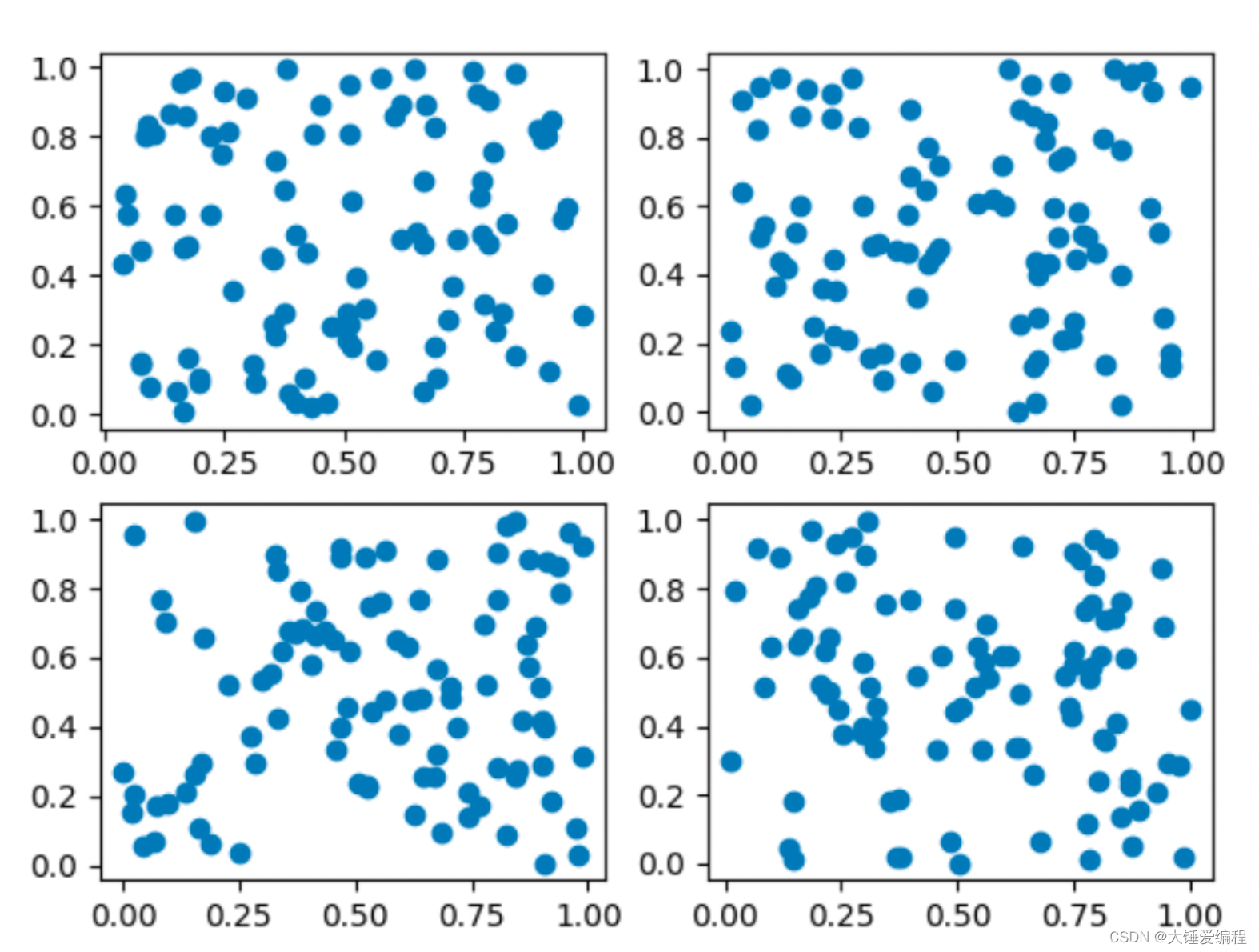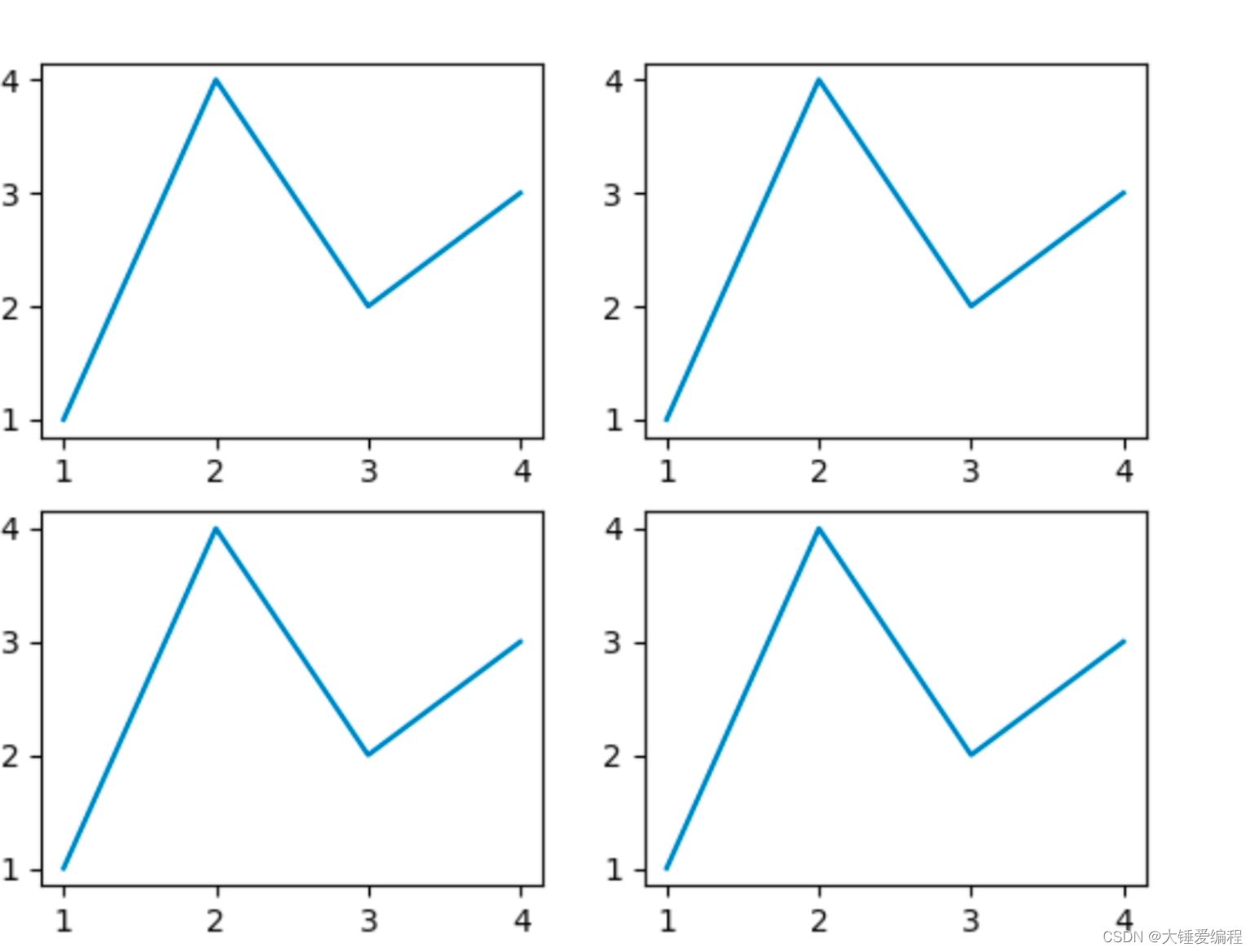# python数据可视化玩转Matplotlib subplot子图操作，四个子图（一包四），三个子图，子图拉伸

1.1 下图是绘制的子图：

1.2 代码释义：

2.1 代码引入

2.2 图形绘制

3.1 子图布局说明

4.1 子图大小调整

5.1 子图代码调整

6.1 代码引入

6.2 完整代码

6.3 完整代码

Matplotlib是一个流行的Python可视化库，它提供了许多功能来创建各种类型的图表。其中一个功能是子图，它允许您在单个图表中绘制多个图。

# 一、创建子图

``````import matplotlib.pyplot as plt

fig, axs = plt.subplots(2, 2)

``````

``````import matplotlib.pyplot as plt
import numpy as np

# 创建一个2x2的网格
fig, axs = plt.subplots(2, 2)

# 在每个子图中绘制一个图形
for ax in axs.flat:
# 随机生成一些数据
x = np.random.rand(100)
y = np.random.rand(100)
# 绘制散点图
ax.scatter(x, y)

# 显示图形
plt.show()

``````

## 1.1 下图是绘制的子图：## 1.2 代码释义：

• 导入必要的库：我们需要使用matplotlib和numpy库来生成散点图和随机数。
• 创建一个2×2的网格：我们使用subplot()函数创建一个2×2的子图，该函数返回一个Figure对象fig和一个Axes对象数组axs，它包含四个子图，第一个参数2表示行数，第二个参数2表示列数。
• 在每个子图中绘制一个图形：我们使用for循环遍历每个子图对象，对每个子图生成随机数据，使用scatter()函数在子图中绘制散点图。使用axs.flat将axs数组展平为一维，这样可以方便地遍历每个子图。
• 随机生成一些数据：我们使用numpy库中的random模块来生成100个在[0,1)内的随机数作为横坐标和纵坐标。
• 绘制散点图：我们使用子图对象ax的scatter()函数来绘制散点图，该函数接受横坐标和纵坐标作为参数，将它们绘制成散点图。
• 显示图形：最后，我们调用plt.show()函数来显示所有子图。这将打开一个窗口，其中包含四个散点图子图。
• # 二、绘制子图

## 2.1 代码引入

绘制子图与绘制普通图形非常相似。您可以使用子图的Axes对象上的任何Matplotlib绘图函数。例如，以下代码将在第一个子图中绘制一条线：

``````import matplotlib.pyplot as plt

fig, axs = plt.subplots(2, 2)
axs[0, 0].plot([1, 2, 3, 4], [1, 4, 2, 3])

``````

要在所有子图中绘制相同的图形，请使用循环。以下代码将在所有子图中绘制一条线：

``````import matplotlib.pyplot as plt

fig, axs = plt.subplots(2, 2)
for ax in axs.flat:
ax.plot([1, 2, 3, 4], [1, 4, 2, 3])

``````

## 2.2 图形绘制

下面是绘制的子图：# 三、子图布局

## 3.1 子图布局说明

默认情况下，`plt.subplots()`函数将子图放置在网格中，每个子图的大小相同。但是，您可以使用各种选项来更改子图的大小和位置。

# 四、子图大小

## 4.1 子图大小调整

要更改子图的大小，请使用`figsize`参数。以下代码将创建一个2×2的网格，其中每个子图的大小为4×4英寸：

``````import matplotlib.pyplot as plt

fig, axs = plt.subplots(2, 2, figsize=(4, 4))

``````

# 五、子图间距

## 5.1 子图代码调整

要更改子图之间的间距，请使用`wspace``hspace`参数。这些参数控制子图之间的水平和垂直间距，以及子图与图表边缘的距离。以下代码将创建一个2×2的网格，其中每个子图的水平和垂直间距为0.5英寸：

``````import matplotlib.pyplot as plt

fig, axs = plt.subplots(2, 2, figsize=(4, 4), wspace=0.5, hspace=0.5)

``````

# 六、子图位置

## 6.1 代码引入

默认情况下，子图将放置在网格中，但您也可以使用`GridSpec`对象来更改子图的位置。以下代码将创建一个网格，其中第一个子图占据整个第一行，而第二个子图占据第一行的后两列：

``````import matplotlib.gridspec as gridspec
import matplotlib.pyplot as plt

fig = plt.figure()
gs = gridspec.GridSpec(2, 2, width_ratios=[1, 2])

``````

## 6.2 完整代码

以下是一个完整的Python代码，演示如何使用`GridSpec`对象来更改子图的位置。该代码将创建一个2×2的网格，其中第一个子图占据整个第一行，而第二个子图占据第一行的后两列。

``````import matplotlib.gridspec as gridspec
import matplotlib.pyplot as plt
import numpy as np

# 创建一个2x2的网格，第一个子图占据整个第一行，第二个子图占据第一行的后两列
gs = gridspec.GridSpec(2, 2, width_ratios=[1, 2])
ax1 = plt.subplot(gs[0, :])
ax2 = plt.subplot(gs[1, 0])
ax3 = plt.subplot(gs[1, 1])

# 在第一个子图中绘制一个折线图
x = np.linspace(0, 10, 100)
y = np.sin(x)
ax1.plot(x, y)

# 在第二个子图中绘制一个散点图
x = np.random.rand(100)
y = np.random.rand(100)
ax2.scatter(x, y)

# 在第三个子图中绘制一个柱状图
x = ['A', 'B', 'C', 'D']
y = [3, 7, 1, 9]
ax3.bar(x, y)

# 显示图形
plt.show()

``````

下面是绘制的子图：## 6.3 完整代码

示例1：使用GridSpec对象创建自定义子图布局

``````import matplotlib.pyplot as plt
import matplotlib.gridspec as gridspec

fig = plt.figure()

# 定义网格
gs = gridspec.GridSpec(3, 3)

# 创建子图1
ax1.set_title('Subplot 1')

# 创建子图2
ax2.set_title('Subplot 2')

# 创建子图3
ax3.set_title('Subplot 3')

# 创建子图4
绘制出来的图片如下，可以看出来，符合实际需求。子图是Matplotlib中强大的功能之一。使用`plt.subplots()`函数，您可以方便地创建多个子图，并使用`Axes`对象绘制各种图形。使用各种选项，例如`figsize``wspace``hspace`参数，以及`GridSpec`对象，可以更改子图的大小、位置和间距。